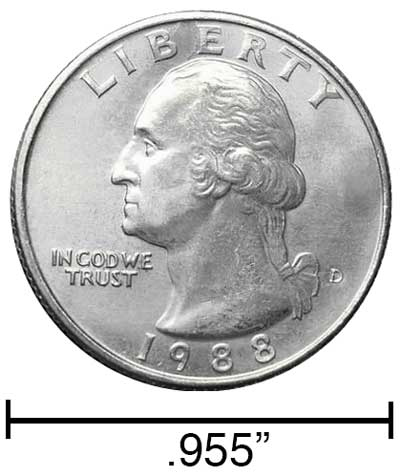# Inches to Nanometers Conversion

Enter the length in inches below to get the value converted to nanometers.

/
Results in Nanometers:1 in = 25,400,000 nm

## How to Convert Inches to NanometersTo convert an inch measurement to a nanometer measurement, multiply the length by the conversion ratio.

Since one inch is equal to 25,400,000 nanometers, you can use this simple formula to convert:

nanometers = inches × 25,400,000

The length in nanometers is equal to the inches multiplied by 25,400,000.

For example, here's how to convert 5 inches to nanometers using the formula above.
5" = (5 × 25,400,000) = 127,000,000 nm

### How Many Nanometers are in an Inch?

There are 25,400,000 nanometers in an inch, which is why we use this value in the formula above.

1" = 25,400,000 nm

Our inch fraction calculator can add inches and nanometers together, and it also automatically converts the results to US customary, imperial, and SI metric values.

## Inches

An inch is a unit of linear length measure equal to 1/12 of a foot or 1/36 of a yard. Because the international yard is legally defined to be equal to exactly 0.9144 meters, one inch is equal to 2.54 centimeters.

The inch is a US customary and imperial unit of length. Inches can be abbreviated as in; for example, 1 inch can be written as 1 in.

Inches can also be denoted using the symbol, otherwise known as a double-prime. Often a double-quote (") is used instead of a double-prime for convenience. A double-prime is commonly used to express 1 in as 1″.The standard ruler has 12", and is a common measuring tool for measuring inches. They are also often measured using tape measures, which commonly come in lengths from 6' - 35'. Other types of measuring devices include scales, calipers, measuring wheels, micrometers, yardsticks, and even lasers.

## Nanometers

One nanometer is equal to one-billionth (1/1,000,000,000) of a meter, which is defined as the distance light travels in a vacuum in a 1/299,792,458 second time interval.

The nanometer, or nanometre, is a multiple of the meter, which is the SI base unit for length. In the metric system, "nano" is the prefix for 10-9. Nanometers can be abbreviated as nm; for example, 1 nanometer can be written as 1 nm.

The nanometer is an extremely small unit of length measurement, often used to measure things that are very small, such as the transistors and electrical pathways in computer processors and nanotechnology.

We recommend using a ruler or tape measure for measuring length, which can be found at a local retailer or home center. Rulers are available in imperial, metric, or combination with both values, so make sure you get the correct type for your needs.

Need a ruler? Try our free downloadable and printable rulers, which include both imperial and metric measurements.

## Inch to Nanometer Conversion Table

Inch measurements converted to nanometers
Inches Nanometers
0.0000001" 2.54 nm
0.0000002" 5.08 nm
0.0000003" 7.62 nm
0.0000004" 10.16 nm
0.0000005" 12.7 nm
0.0000006" 15.24 nm
0.0000007" 17.78 nm
0.0000008" 20.32 nm
0.0000009" 22.86 nm
0.00000001" 0.254 nm
0.0000001" 2.54 nm
0.000001" 25.4 nm
0.00001" 254 nm
0.0001" 2,540 nm
0.001" 25,400 nm
0.01" 254,000 nm
0.1" 2,540,000 nm
1" 25,400,000 nm

## References

1. National Institute of Standards and Technology, Checking the Net Contents of Packaged Goods, Handbook 133 - 2019 Edition, https://nvlpubs.nist.gov/nistpubs/hb/2019/NIST.HB.133-2019.pdf